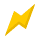115 Volts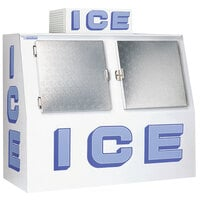\$2,489.00/Each115 Volts\$2,599.00/Each115 Volts\$2,039.00/Each115 Volts\$2,139.00/Each115 Volts\$1,929.00/Each115 Volts\$3,179.00/Each115 Volts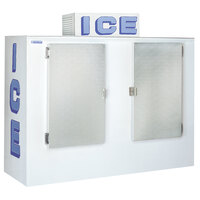\$3,029.00/Each115 Volts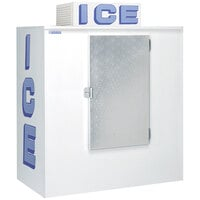\$2,489.00/Each115 Volts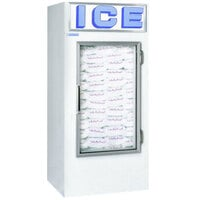\$2,389.00/Each115 Volts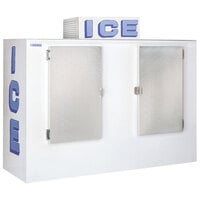\$3,439.00/Each

\$1,989.00/Each

\$1,979.00/Each

\$2,729.00/Each

\$2,319.00/Each

\$1,869.00/Each

\$3,539.00/Each

\$4,739.00/Each115 Volts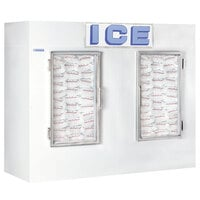\$4,529.00/Each